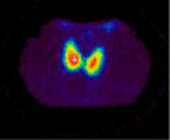jörg piringer - digital sound visual interactive poetry etc.
hpr

hyperthyreosisa mini release about hyperthyreosis (or rather about some terms of this illness). it was done with a casio PT-7 through circuit bending with body contact only. the sound was then processed with a computer.

v
r
z
p
b
s
r
d
y
b
c
p
y
t
z
a
v
h
m
x
f
s
v
w
p
c
l
x
l
h
b
f
b
y
b
f
i
c
s
a
w
q
w
e
p
h
s
o
c
c
c
r
p
k
s
v
s
f
w
a
w
k
i
y
x
p
z
f
g
q
u
s
e
t
d
h
s
b
w
m